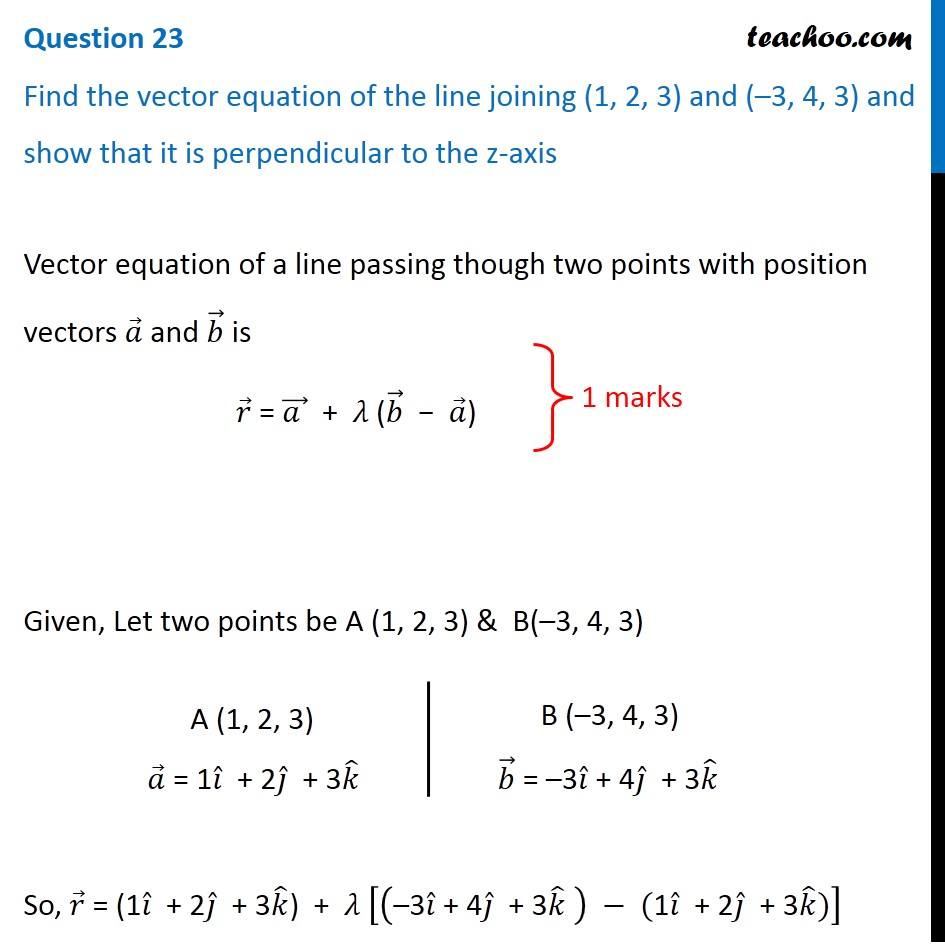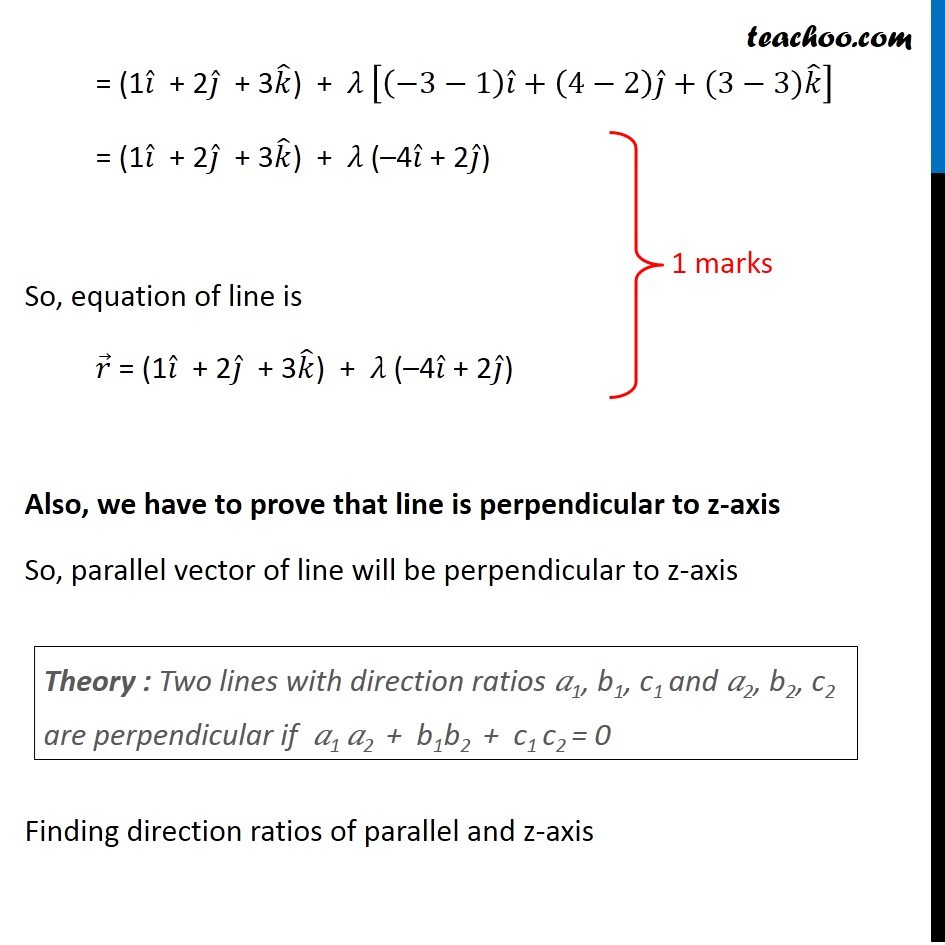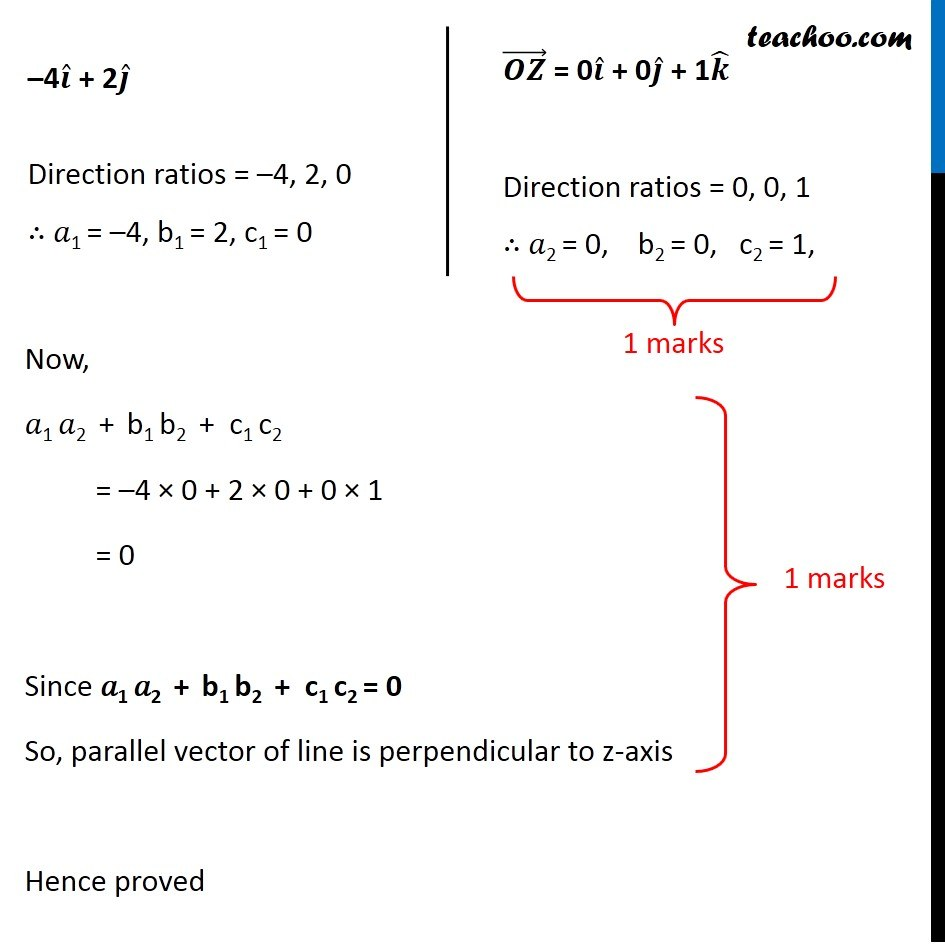CBSE Class 12 Sample Paper for 2019 Boards

Class 12
Solutions of Sample Papers and Past Year Papers - for Class 12 Boards

Question 23

Find the vector equation of the line joining (1, 2, 3) and (–3, 4, 3) and show that it is perpendicular to the z-axisLearn in your speed, with individual attention - Teachoo Maths 1-on-1 Class

### Transcript

Question 23 Find the vector equation of the line joining (1, 2, 3) and (–3, 4, 3) and show that it is perpendicular to the z-axis Vector equation of a line passing though two points with position vectors 𝑎 ⃗ and 𝑏 ⃗ is 𝑟 ⃗ = (𝑎 ) ⃗ + 𝜆 (𝑏 ⃗ − 𝑎 ⃗) Given, Let two points be A (1, 2, 3) & B(–3, 4, 3) A (1, 2, 3) 𝑎 ⃗ = 1𝑖 ̂ + 2𝑗 ̂ + 3𝑘 ̂ B (–3, 4, 3) 𝑏 ⃗ = –3𝑖 ̂ + 4𝑗 ̂ + 3𝑘 ̂ So, 𝑟 ⃗ = (1𝑖 ̂ + 2𝑗 ̂ + 3𝑘 ̂) + 𝜆 [("–3" 𝑖 ̂" + 4" 𝑗 ̂" + 3" 𝑘 ̂" " ) − ("1" 𝑖 ̂" + 2" 𝑗 ̂" + 3" 𝑘 ̂)] = (1𝑖 ̂ + 2𝑗 ̂ + 3𝑘 ̂) + 𝜆 [(−3−1) 𝑖 ̂+(4−2) 𝑗 ̂+(3−3)𝑘 ̂ ] = (1𝑖 ̂ + 2𝑗 ̂ + 3𝑘 ̂) + 𝜆 (–4𝑖 ̂ + 2𝑗 ̂) So, equation of line is 𝑟 ⃗ = (1𝑖 ̂ + 2𝑗 ̂ + 3𝑘 ̂) + 𝜆 (–4𝑖 ̂ + 2𝑗 ̂) Also, we have to prove that line is perpendicular to z-axis So, parallel vector of line will be perpendicular to z-axis Theory : Two lines with direction ratios 𝑎1, b1, c1 and 𝑎2, b2, c2 are perpendicular if 𝑎1 𝑎2 + b1b2 + c1 c2 = 0 Finding direction ratios of parallel and z-axis –4𝒊 ̂ + 2𝒋 ̂ Direction ratios = –4, 2, 0 ∴ 𝑎1 = –4, b1 = 2, c1 = 0 (𝑶𝒁) ⃗ = 0𝒊 ̂ + 0𝒋 ̂ + 1𝒌 ̂ Direction ratios = 0, 0, 1 ∴ 𝑎2 = 0, b2 = 0, c2 = 1, Now, 𝑎1 𝑎2 + b1 b2 + c1 c2 = –4 × 0 + 2 × 0 + 0 × 1 = 0 Since 𝑎1 𝑎2 + b1 b2 + c1 c2 = 0 So, parallel vector of line is perpendicular to z-axis Hence proved